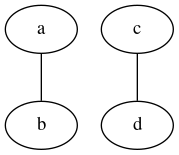# Graphviz dot简明教程

graphviz是一个用特殊的文本语言来生成图片的应用，可以生成有向图和无向图。dot就是那个特殊的文本语言。

dot大概长这样：

``````graph {
a -- b;
c -- d;
}
``````

``````\$ dot -Tpng -o dot_demo.png dot_demo.dot
``````## 安装

``````\$ sudo pacman -S graphviz
``````

## 语法

``````graph graph_name {
a -- b;
c -- d;
}
``````

`graph_name` 是可选的，如果没有，就是一个匿名图。其中，`a`, `b`, `c`, `d` 都是一个节点，可以使用 `node` 关键字来进行属性设定，例如上面的变成：

``````graph graph_name {
node [color=Red];

a -- b;
c -- d;
}
``````• `node`: 修饰节点
• `edge`: 修饰连线

• `bgcolor`: 背景颜色
• `shape`: 形状
• `size`: 大小

## 标注和变量声明

``````digraph graph_name {
T [label="Teacher" color=Blue, fontcolor=Red, fontsize=24, shape=box]      // node T
P [label="Pupil" color=Blue, fontcolor=Red, fontsize=24, shape=box]  // node P

T->P [label="Instructions", fontcolor=darkgreen] // edge T->P
}
``````##### 更多文章
• Linux常用命令(一)：netcat
• 结合Flask 与 marshmallow快速进行参数校验
• 规整数据的重要性
• apt安装特定包以及忽略升级某个包
• Goroutine是如何处理栈的？
• StackGuard的作用
• Go DiskQueue源码阅读
• NSQ源码分析
• NSQ简明教程
• 结合Redis与MySQL实现又快又好的数据方案
• 程序员的MySQL手册(五)：索引优化
• 程序员的MySQL手册(四)：索引设计
• 程序员的MySQL手册(三)：数据库设计
• Linux窗口管理器下的截图
• Go设计模式：facade模式和观察者模式

•本站热门